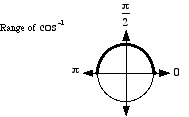index: click on a letter A B C D E F G H I J K L M N O P Q R S T U V W X Y Z A to Z index index: subject areas numbers & symbols sets, logic, proofs geometry algebra trigonometry advanced algebra & pre-calculus calculus advanced topics probability & statistics real world applications multimedia entrieswww.mathwords.com about mathwords website feedback

 Inverse Cosine cos-1 Cos-1 arccos Arccos The inverse function of cosine. Basic idea: To find cos-1 (½), we ask "what angle has cosine equal to ½?" The answer is 60°. As a result we say cos-1 (½) = 60°. In radians this is cos-1 (½) = π/3. More: There are actually many angles that have cosine equal to ½. We are really asking "what is the simplest, most basic angle that has cosine equal to ½?" As before, the answer is 60°. Thus cos-1 (½) = 60° or cos-1 (½) = π/3. Details: What is cos-1 (–½)? Do we choose 120°, –120°, 240°, or some other angle? The answer is 120°. With inverse cosine, we select the angle on the top half of the unit circle. Thus cos-1 (–½) = 120° or cos-1 (–½) = 2π/3. In other words, the range of cos-1 is restricted to [0, 180°] or [0, π]. Note: arccos refers to "arc cosine", or the radian measure of the arc on a circle corresponding to a given value of cosine. Technical note: Since none of the six trig functions sine, cosine, tangent, cosecant, secant, and cotangent are one-to-one, their inverses are not functions. Each trig function can have its domain restricted, however, in order to make its inverse a function. Some mathematicians write these restricted trig functions and their inverses with an initial capital letter (e.g. Cos or Cos-1). However, most mathematicians do not follow this practice. This website does not distinguish between capitalized and uncapitalized trig functions.See also